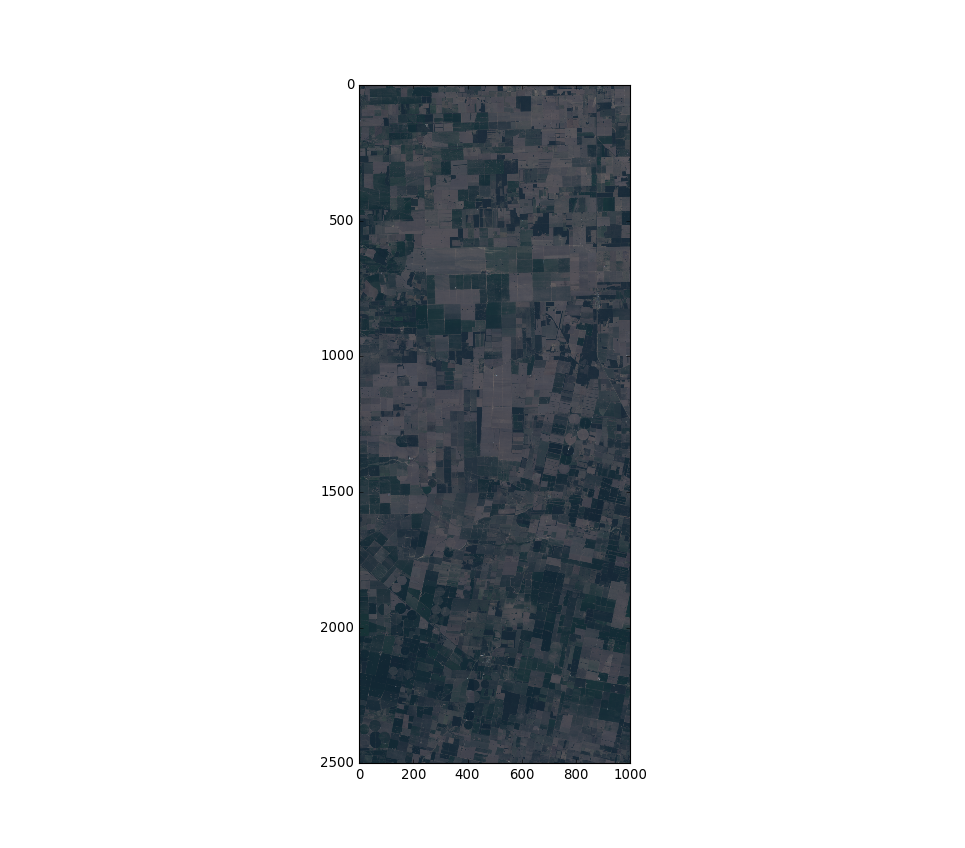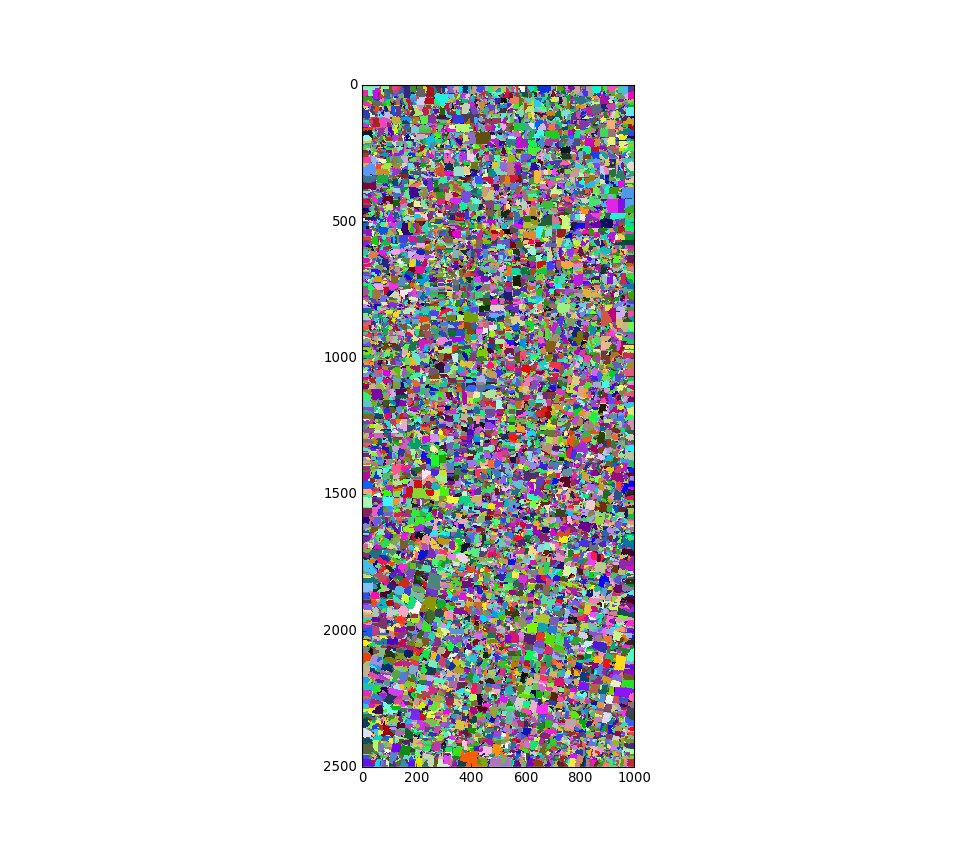# OBIA in Python¶

This notebook is support material for the blog post here: http://www.machinalis.com/blog/obia/

The code in the blog has been simplified so it may differ from what's done here.

In :
%matplotlib notebook

import numpy as np
import os
import scipy

from matplotlib import pyplot as plt
from matplotlib import colors
from osgeo import gdal
from skimage import exposure
from skimage.segmentation import quickshift, felzenszwalb
from sklearn import metrics
from sklearn.ensemble import RandomForestClassifier

RASTER_DATA_FILE = "data/image/2298119ene2016recorteTT.tif"
TRAIN_DATA_PATH = "data/train/"
TEST_DATA_PATH = "data/test/"


First, define some helper functions (taken from http://www.machinalis.com/blog/python-for-geospatial-data-processing/)

In :
def create_mask_from_vector(vector_data_path, cols, rows, geo_transform,
projection, target_value=1):
"""Rasterize the given vector (wrapper for gdal.RasterizeLayer)."""
data_source = gdal.OpenEx(vector_data_path, gdal.OF_VECTOR)
layer = data_source.GetLayer(0)
driver = gdal.GetDriverByName('MEM')  # In memory dataset
target_ds = driver.Create('', cols, rows, 1, gdal.GDT_UInt16)
target_ds.SetGeoTransform(geo_transform)
target_ds.SetProjection(projection)
gdal.RasterizeLayer(target_ds, , layer, burn_values=[target_value])
return target_ds

def vectors_to_raster(file_paths, rows, cols, geo_transform, projection):
"""Rasterize all the vectors in the given directory into a single image."""
labeled_pixels = np.zeros((rows, cols))
for i, path in enumerate(file_paths):
label = i+1
ds = create_mask_from_vector(path, cols, rows, geo_transform,
projection, target_value=label)
band = ds.GetRasterBand(1)
ds = None
return labeled_pixels

In :
raster_dataset = gdal.Open(RASTER_DATA_FILE, gdal.GA_ReadOnly)
geo_transform = raster_dataset.GetGeoTransform()
proj = raster_dataset.GetProjectionRef()
n_bands = raster_dataset.RasterCount
bands_data = []
for b in range(1, n_bands+1):
band = raster_dataset.GetRasterBand(b)

bands_data = np.dstack(b for b in bands_data)


Create images

In :
img = exposure.rescale_intensity(bands_data)
rgb_img = np.dstack([img[:, :, 3], img[:, :, 2], img[:, :, 1]])

In :
plt.figure()
plt.imshow(rgb_img)Out:
<matplotlib.image.AxesImage at 0x7fbeeff0d0f0>
In :
segments_quick = quickshift(img, kernel_size=7, max_dist=3, ratio=0.35, convert2lab=False)
n_segments = len(np.unique(segments_quick))
print(n_segments)

26792

In :
cmap = colors.ListedColormap(np.random.rand(n_segments, 3))
plt.figure()
plt.imshow(segments_quick, interpolation='none', cmap=cmap)Out:
<matplotlib.image.AxesImage at 0x7fbeefe5d400>

skimage.segmentation.felzenszwalb is not prepared to work with multi-band data. So, based on their own implementation for RGB images, I apply the segmentation in each band and then combine the results. See: http://github.com/scikit-image/scikit-image/blob/v0.12.3/skimage/segmentation/_felzenszwalb.py#L69

In :
band_segmentation = []
for i in range(n_bands):
band_segmentation.append(felzenszwalb(img[:, :, i], scale=85, sigma=0.25, min_size=9))


put pixels in same segment only if in the same segment in all bands. We do this by combining the band segmentation to one number

In :
const = [b.max() + 1 for b in band_segmentation]
segmentation = band_segmentation
for i, s in enumerate(band_segmentation[1:]):
segmentation += s * np.prod(const[:i+1])

_, labels = np.unique(segmentation, return_inverse=True)
segments_felz = labels.reshape(img.shape[:2])

In :
cmap = colors.ListedColormap(np.random.rand(len(np.unique(segments_felz)), 3))
plt.figure()
plt.imshow(segments_felz, interpolation='none', cmap=cmap)